# Exclusive disjunction facts for kids

Kids Encyclopedia Facts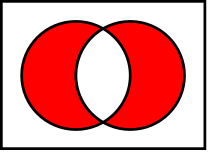Venn diagram of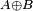$A \oplus B$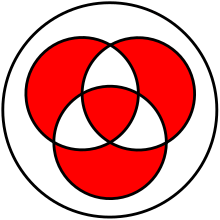Venn diagram of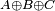$A \oplus B \oplus C$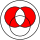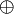$~\oplus~$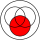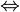$~\Leftrightarrow~$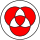Exclusive disjunction (also called exclusive or, XOR) is a logic operation on two values. It is often represented by the symbol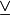$\veebar$ (or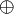$\oplus$). It will be true, if exactly one of the two values is true. Otherwise, it will be false. This also means that the result of 'XOR' will be true precisely both the values are different. Same values will result in a false.

The best way to remember a XOR operation is: "One or the other, but not both".

Because of that, this is different from inclusive disjunction.

## Truth table

The truth table of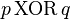$p\, \mathrm{XOR}\, q$ (also written as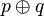$p \oplus q$,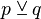$p \veebar q$, or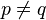$p \neq q$) is as follows:

p q
F F F
F T T
T F T
T T F

## Related pagesExclusive disjunction Facts for Kids. Kiddle Encyclopedia.